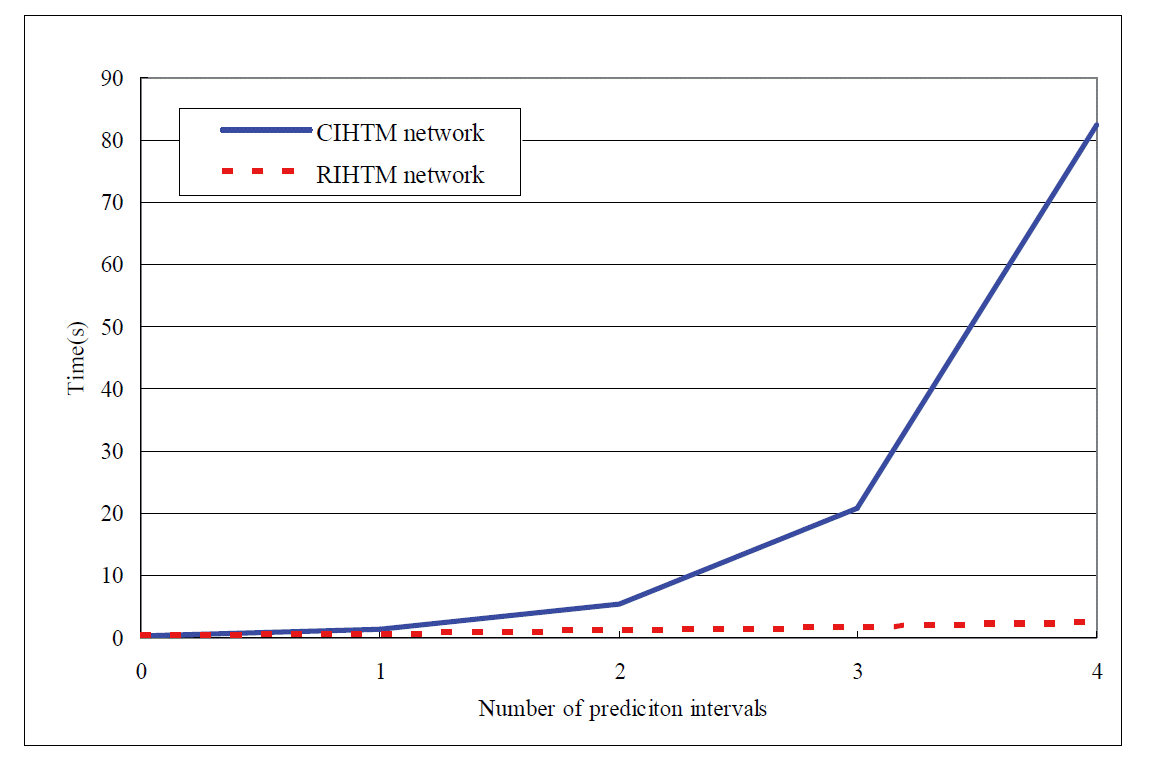Kang: A Real-Time Integrated Hierarchical Temporal Memory Network for the Real-Time Continuous Multi-Interval Prediction of Data Streams

### Abstract

Continuous multi-interval prediction (CMIP) is used to continuously predict the trend of a data stream based on various intervals simultaneously. The continuous integrated hierarchical temporal memory (CIHTM) network performs well in CMIP. However, it is not suitable for CMIP in real-time mode, especially when the number of prediction intervals is increased. In this paper, we propose a real-time integrated hierarchical temporal memory (RIHTM) network by introducing a new type of node, which is called a Zeta1FirstSpecializedQueueNode (ZFSQNode), for the real-time continuous multi-interval prediction (RCMIP) of data streams. The ZFSQNode is constructed by using a specialized circular queue (sQUEUE) together with the modules of original hierarchical temporal memory (HTM) nodes. By using a simple structure and the easy operation characteristics of the sQUEUE, entire prediction operations are integrated in the ZFSQNode. In particular, we employed only one ZFSQNode in each level of the RIHTM network during the prediction stage to generate different intervals of prediction results. The RIHTM network efficiently reduces the response time. Our performance evaluation showed that the RIHTM was satisfied to continuously predict the trend of data streams with multi-intervals in the real-time mode.

### 1. Introduction

The prediction of data streams has become one of the most challenging problems in the area of pattern recognition . Many researchers have tried to build systems for effectively predicting the trend of data streams . These systems are constructed to predict the trend of data streams by using various base technologies. For example, Lee and Jo  used an expert system and Angelov and Zhou  used a fuzzy system. The continuous multi-interval prediction (CMIP) technology among of all is used to predict the trend of a data stream based on various intervals without interruption. It is important to support the CMIP so that it is able to satisfy different prediction requirements effectively. Therefore, the continuous integrated hierarchical temporal memory (CIHTM) network has been suggested as a means to run the CMIP technology . However, it is not suitable for real-time mode, especially when the number of prediction intervals is increased. It is necessary to provide CMIP in the real-time mode because data streams are continuously generated in a dynamic environment .
We are proposing a real-time integrated hierarchical temporal memory (RIHTM) network for real-time continuous multi-interval prediction (RCMIP) by extending the CIHTM network. The RIHTM network is constructed using a new type of node, which is called a Zeta1FirstSpecialized QueueNode (ZFSQNode). This node is composed of a specialized circular queue (sQUEUE) and the modules of original hierarchical temporal memory (HTM) nodes [8,9]. By using a simple structure and the easy operation characteristics of the sQUEUE, the ZFSQNode aggregates all of the prediction information together and processes all of the prediction tasks in the data structure of it. In particular, each level of the RIHTM network contains only one ZFSQNode at the prediction stage. Because the amount of nodes in each level is not changed while the interval length for predicting trends increase, the RIHTM network reduces memory and time consumption effectively. In addition, we employed a real-time input sensor (StreamDataSensor) to then input data into the ZFSQNode in real-time mode. The StreamDataSensor assists the ZFSQNode in generating prediction results in the real-time mode.
This paper is organized in 6 sections. Section 2 describes the CIHTM network. In Section 3, we propose the RIHTM network for RCMIP. Section 4 describes training and prediction in the RIHTM network with the new node. In Section 5, we present the comparison results between two networks and give a performance evaluation. Finally, we conclude this paper in Section 6.

### 2. CIHTM Network

In this section, we briefly review the CIHTM network for CMIP and show its efficiency issues.

### 2.1 CMIP Concepts

A data stream can be regarded as a sequence of attribute value vectors. The vector items based on a different time granularity form a continuous flow of data like a stream. It maps each time point to a numeric value. The prediction for a data stream is to decide the trend of the changing movement based on its past behaviors.
In Fig. 1(a), the left side shows a diagram for a data stream s(t): t ∈ [t0, t5], where t0 is the starting time and t5 is the ending time (current time). Traditional prediction methods  are based on a fixed length of the time interval of historical data in the continuous mode. For example, take the data stream that is shown on the left side of Fig. 1(a). In this case traditional prediction methods can only provide a single prediction result at the current time t5 of a fixed time (in this example it was 5 time units) interval from t0 to t5. At time t11, a new value arrives and a single prediction result of a fixed time interval from t6 to t11 is generated, as shown on the right side of Fig. 1(a).
Although the traditional prediction methods for a fixed time interval work well, many applications require multiple prediction results that are based on different time intervals for satisfying diverse requirements. The multi-interval prediction (MIP) has been proposed for predicting the trend of a data stream based on various intervals of historical data . If you look at the data stream shown on the left side of Fig. 1(a) you will see that the MIP can provide three prediction results at the current time t5 of three time intervals from t4 to t5, t2 to t5, t0 to t5 simultaneously, as shown in the right side of Fig. 1(b). MIP can effectively satisfy the diverse requirements of different users. However, MIP neglects most of the important characteristics of timeliness and continuity in predicting data streams. As shown on the right side of Fig. 1(b), MIP does not provide a prediction at subsequence time point t6, but it does provide three prediction results at t11 of three time intervals from t10 to t11, t8 to t11, t6 to t11 simultaneously. As such, we lose the prediction information from t6 to t10 because the HTM is restricted in predicting the trend of data streams discontinuously.
In order to develop of a timely, continuous, and multi-interval prediction tool, a new prediction method continuous multi-interval prediction (CMIP) is proposed by Kang and Diao .
The CMIP combines the two concepts of continuous prediction and multi-interval prediction. It attempts to continuously predict the trend of data streams based on multiple intervals simultaneously. As shown on the left side of Fig. 1(c), the CMIP provides three prediction results at the current time t5 of three time intervals from t4 to t5, t2 to t5, t0 to t5 simultaneously. It continuously generates three prediction results at the subsequence time point t6 of t5, as shown on the right side of Fig. 1(c).

### 2.2 The CIHTM Network for CMIP

The CIHTM network is constructed with three types of nodes (Zeta1Node, Zeta1FirstNode, and Zeta1TopNode), together with the VectorFileSensor, VectorFileEffector, and MultipleOutput Effector. The first position of each level uses the Zeta1FirstNode, where the remaining locations use the Zeta1Node. The top level has only one Zeta1TopNode.
The CIHTM network works in two stages—training and prediction . The CIHTM network is based on the HTM network whose training and prediction algorithms are described by George and Jaros 
During the training stage, only the Zeta1FirstNode at each level and the Zeta1TopNode at the top level perform supervised learning based on the category information (shown in Fig. 1). In Fig. 2(a), the nodes in level 1, level 2, and the top level process the data streams with 1-minute, 4-minute, and 12-minute intervals, respectively. After training, the network can be used to perform predictions. During the prediction stage, the ShiftVectorFileSensor is used in the bottom of the hierarchy for continuously inputting data to the network. The prediction results based on 1-minute, 4-minute, and 12-minute intervals are generated by the Zeta1FirstNode at level 1, level 2, and the Zeta1TopNode at the top level, as shown in Fig. 2(b). The MultipleOutputEffector at the top of the hierarchy receives prediction results from different levels simultaneously and determines whether these outputs are valid.

### 2.3 Efficiency Issues of the CIHTM Network in Real-Time Prediction

The CIHTM network performs well in CMIP . However, the CIHTM network is not suitable for making a real-time prediction, because it is suboptimal for reducing time consumption at the prediction stage. The efficiency problems of the CIHTM network in real-time prediction are analyzed as laid out below.
First, in the CIHTM network, every time new data item arrives, the item is not directly input into the nodes for prediction. Instead the item is stored in the leftmost part of the ShiftVectorFileSensor and all the existing data in the sensor is concurrently shifted to the right. Then, the updated input data of the sensor is uploaded to the corresponding nodes at the bottom level. The processes of storing, right shifting, and uploading in the ShiftVectorFileSensor have spent long time to treat stream data before the network receives and produces prediction results. As a result, the CIHTM network cannot receive data in the real-time mode.
Second, in terms of the entire network during the prediction stage, the amount of nodes from a certain level is decided by predicting capability of the level or in other words, by the interval lengths of predicting. As shown in Fig. 2, the network is able to predict data streams of 12-minute intervals with a time granularity of 1 minute, and it needs 12 homogeneous nodes to process the data streams in the first level. Therefore, if we want to predict a data stream with a 100-minute interval we need 100 nodes in the first level, which results in high memory and time consumption.
Third, although the data is inputted into the nodes simultaneously, the operation of each node in the same level is in successive order. For instance, in the first level, the second node is only available when the first node completes its operation. In the same way, all of the nodes are activated in sequence to perform predictions one-by-one and level-to-level. This mechanism results in an increase in time consumption as the interval lengths of predicting increases.
It is crucial to develop an effective approach to provide timely predictions for real-time data with a lower overhead. We will propose a feasible approach in the next two sections.

### 3. The RIHTM Network for RCMIP

As mentioned above, the utilization of redundant homogenous nodes of the CIHTM network results in excessive memory and time consumption. In order to simplify the operation and structure of the network, we propose a new type of node, the Zeta1FirstSpecializedQueueNode (ZFSQNode), to construct a RIHTM network. In this section, we describe the ZFSQNode and the RIHTM network.

### 3.1 ZFSQNode

The ZFSQNode is an extended node of Zeta1FirstNode with an additional specialized circular queue, where the Zeta1FirstNode integrates the properties of both Zeta1Node and Zeta1TopNode . The structure of ZFSQNode is shown in Fig. 3.
The ZFSQNode is used to process the current data item of real-time data streams at the prediction stage. The arriving data item is input into the spatial pooler, and then the output of the spatial pooler is sent to the supervised mapper and temporal pooler simultaneously. The supervised mapper generates the prediction result of the current node for the arriving data item. The specialized circular queue as a memory stores the outputs of the temporal pooler for the previous data items in each unit cell. The combination of the output of the temporal pooler for the current data item and the outputs of the queue as an output of the ZFSQNode is uploaded to its parent node.

### 3.2 Specialized Circular Queue of the ZFSQNode

The sQUEUE in the ZFSQNode is a fix-sized memory buffer. It is a circular queue in which the multiple values that are located in the middle positions are outputted instead of a value that is located at the front position. We used an insert pointer to represent the position of inserting data in sQUEUE. A key advantage of sQUEUE is that it does not need to be shuffled around when multiple data from the queue are read out. This means that the computational cost of output is independent of the size of the queue and the amount of readout data. In Fig. 4, an initial sQUEUE of ZFSQNode is shown.
The insertpointer represents the position of inserting data in the sQUEUE. Size identifies the length of the queue; Readoutnum and Position identify how much data needs to be readout and the position of each piece of readout data, respectively. The Size, Readoutnum, and the Position of the sQUEUE of ZFSQNode are different at each level in the RIHTM network.
The Size depends on the interval length of the processing data of the ZFSQNode at a higher level. The queue size of level i, labeled Sizei, is computed as:
##### (1)
Sizei=Li+1-1
where Li is the interval length of the processing data of ZFSQNode at level i.
The Readoutnum depends on the number of sibling nodes of this node. The readout number of level i, labeled Readoutnumi, is computed as:
##### (2)
where Childnodenumi is the number of child nodes of ZFSQNode at level i.
The jth readout data position of the queue at level i from the insertpointer, labeled Positioni[j], is computed as:
##### (3)
Positioni[j]=(Li+1/Childnodenumi+1-1)+Li×(j-1)
where 1 ≤ j ≤ Readoutnumi.
According to the dynamic data streams management, the frequent changes with the readout positions and multiple readout data from the queue are challenges to be solved. However, the sQUEUE is very easy to operate as it contains only three operations: create, readout, and insert. Create a sQUEUE. The sQUEUE first starts empty and has a calculated queue size, readout number, and position. The insert pointer points to the cell with an index of 0.
1. Readout data in all positions: this reads out data from the queue using calculated positions.

2. Insert data: this inserts data and moves the insert pointer to the next cell. The insert pointer moves in a circle along the circular queue.

### 3.3 Structure of RIHTM Network

Fig. 5 shows a structure with three simple levels in the RIHTM network for RCMIP. The network has three types of nodes (Zeta1Node, ZFSQNode, and Zeta1TopNode), along with the VectorFileSensor, VectorFileEffector, and MultipleOutputEffector.
In Fig. 5, a data stream is inputted to the network and fed to the specified nodes at the bottom level. The input to the network is a data stream with a 12-minute interval with a time granularity of 1 minute. The data items in the data stream are recorded every other interval and are inputted into the corresponding node at the bottom level of the network. The inputs to the nodes at level 2 take place every 4 minutes interval. The Zeta1TopNode at the top level takes place over the entire 12-minute interval.
At each level, there is only one ZFSQNode. The ZFSQNode is located in the first position, where the remaining locations use the Zeta1Nodes. The top level has only one Zeta1TopNode. The ZFSQNode and the Zeta1TopNode are used to perform supervised learning and prediction based on the category information from the VectorFileSensor (Category Data).
The VectorFileEffector at the corresponding level receives the outputs from the ZFSQNode and the Zeta1TopNode. Then, the output from the VectorFileEffector at each level is sent to the MultipleOutputEffector. Meanwhile, the MultipleOutputEffector determines whether the output is valid and generates the MIP results.

### 4. Training and Prediction of the RIHTM Network

In this section, we describe how the RIHTM network operated in the training and prediction stages with the assistance of ZFSQNode.

### 4.1 Training Stage

The RIHTM network performs training level-by-level. Fig. 6 shows the operating procedures of the network during the training stage.
In the first step of training (➀), as shown in Fig. 6, a historical data stream with data items recorded per minute (a data stream with a 1-minute interval) is input into the network. Only the ZFSQNode at level 1 is enabled to receive data items from the VectorFileSensor and gets into the learning mode. After all the learning data has been represented, the structures of the spatial pooler and the temporal pooler in the ZFSQNode are copied to the Zeta1Nodes at the same level. Entering into Step 2 (➁) shown in Fig. 6, the ZFSQNode at level 2 gets into the learning mode. Four child nodes (ZFSQ and 3 Zeta1Nodes) at level 1 get into the inference mode. The ZFSQNode at level 2 receives the learning data stream from its four child nodes at a 4-mimute interval. After the ZFSQNode at level 2 has learned all of the data, the data structures are copied to the remaining Zeta1Nodes at level 2 as with the ZFSQNode at level 1. This procedure is repeated throughout the hierarchy until all of the nodes in the network are enabled. As shown in Fig. 6, the Zeta1TopNode at the top level is put into the learning mode (➂). Its 3 child nodes at level 2 and 12 grandchild nodes at level 1 perform inference. The inference outputs of the nodes at level 1 are sent to the nodes at level 2, and then the inference outputs of the nodes at level 2 are sent to the Zeta1TopNode as learning data. The Zeta1TopNode is trained using the data stream at a 12-minute interval. During the whole training stage, the sQUEUE is disabled as it is used only in the prediction stage.

### 4.2 Prediction Stage

During the prediction stage, the RIHTM network uses an advanced prediction technique for RCMIP. Fig. 7 shows the structure of the RIHTM network for the prediction stage. It is obvious that the RITHM network has a simpler structure than the CIHTM network (depicted in Fig. 2) and that it is faster and easier to operate.
During the prediction stage, the data that is input into the trained network is a dynamic sequence of real-time data. To generate prediction results in the real-time mode, we used a StreamDataSensor to process real-time data streams in a timed sequence. StreamDataSensor plays the same role as the VectorFileSensor in the training stage. The only difference between them is that the StreamDataSensor contains only one sensor for real-time input data streams with a fixed interval (1 minute).
The ZFSQNode with its sQUEUE in each level and the Zeta1TopNode at the top level are enabled to expose new data with 1-minute, 4-minute, and 12-minute intervals, respectively, and to produce the prediction results for these three intervals simultaneously. The prediction results of these three intervals are received by the MultipleOutputEffector to determine whether these outputs are valid, and the MultipleOutputEffector generates the multi-interval prediction results of the network.
Fig. 8 shows different steps of the RIHTM network during the prediction stage.
In Fig. 8, the ZFSQNode at level 1 receives the sequence of input data with the 1-minute interval from StreamDataSensor. The input data vi at ti is the value difference from ti-1 to ti. For instance, if the value is 8.01 at ti-1 and the value is 8.12 at ti, then the difference value in the interval (i-1)-i from ti-1 to ti, vi is +0.11. The spatial pooler of the ZFSQNode compares this input vector to each coincidence that was learned during the training stage and computes a belief vector for its input vector. This belief vector is sent to the temporal pooler and supervised mapper simultaneously. The supervised mapper of the ZFSQNode receives this belief vector and produces a distribution over the categories as the 1-minute interval prediction result of level 1. Meanwhile, the temporal pooler receives the belief vector from the spatial pooler and calculates belief distribution over groups of intervals (i-1)-i, which is marked as p(i-1) - i. The p(i-1) - i is a vector that is the same size as the number of temporal groups. For example, the node has 12 coincidences within its spatial pooler and four temporal groups within its temporal pooler. The present input matches the fourth coincidence, which belongs to temporal group 2. Therefore, the output of the temporal pooler p(i-1) - i is a vector of four groups with one match corresponding to group 2 and is represented as . The output p(i-1) - i of the temporal pooler is concatenated with the outputs of sQUEUE. The Size, Readoutnum, and Position of sQUEUE at level 1 in Fig. 8 is 3, 3, [0, 1, 2], respectively. If there is no data in the sQUEUE, each output of the queue is set to the default value 0. Therefore, the concatenation of the output from the temporal pooler and sQUEUE is p0-1, 0, 0, 0, which is the input of the ZFSQNode at level 2.
This procedure is repeated up the hierarchy until the outputs of all the levels are valid. These multiple prediction results are used to predict the real-time data stream trends at the current time according to different intervals.

### 5. Performance Analysis

The RIHTM network is suitable for RCMIP. It is effective in tremendously reducing memory and time consumption when the number of prediction intervals is increased. In the following section, the performance analysis of memory and time consumption is discussed.

### 5.1 Analytical Expression

In this section we will describe the analytical expression for the estimation of memory and time consumption in the CITHM network and RIHTM network at the prediction stage.

#### 5.1.1 Estimation of memory consumption

The spatial pooler, temporal pooler, and the supervised mapper are the key substructures of nodes that perform learning and inference. The spatial pooler analyzes the stream of input patterns and generates a coincidence matrix with a row for each coincidence and one column for each element in the input pattern. The memory consumption of spatial pooler is mSP (ncoincs, mpatterns) = ncoincs × mpattern, where ncoincs is the number of coincidences generated by the spatial pooler and mpattern denotes the memory of an input pattern. The temporal pooler receives coincidence indices that are sent by the spatial pooler and forms non-overlapping groups of coincidences using a time-adjacency matrix with ncoincs rows and ncoincs columns. The memory consumption of temporal pooler is mTP (ncoincs) = ncoincs × ncoincs + ncoincs. The supervised mapper does not form groups, but instead forms a matrix with ncoincs rows and one column for each category that it encountered from the category sensor. The memory consumption of the supervised mapper is mSM (ncategs, ncoincs) = ncategs × ncoincs, where the ncategs denotes the number of categories.
A Zeta1Node consists of a spatial pooler and a temporal pooler. Therefore, the memory consumption of a Zeta1Node is mZ1 = mSP + mTP = (ncoincs × mpattern) + (ncoincs × ncoincs + ncoincs). A Zeta1FirstNode consists of a spatial pooler, a temporal pooler, and a supervised mapper. Therefore, the memory consumption of a Zeta1FirstNode is mZF = mSP + mTP + mSM = (ncoincs × mpattern) + (ncoincs × ncoincs × mindex + ncoincs × mindex) + (ncategs ×ncoincs × mindex). A Zeta1TopNode consists of a spatial pooler and a supervised mapper. The memory consumption of a Zeta1TopNode is mZT = mSP + mSM = (ncoincs × mpattern) + (ncategs ×ncoincs × mindex).
The CIHTM network is constructed with all of these three types of nodes at the prediction stage. In the network, there is only one Zeta1FirstNode in each level and it always located in the first position and the remaining locations use the Zeta1Nodes. However, there is an exception where the top level has only one Zeta1TopNode. Therefore, the memory consumption of the CIHTM network with n intervals prediction at the prediction stage can be calculated by the following formula:
##### (4)
MC=mZT+i=1n-1mZF(i)+i=1n-1(nZ1(i)×mZ1(i))
where mZT is the memory consumption of Zeta1TopNode; mZF(i) is the memory consumption of Zeta1FirstNode at level i; mZ1(i) is the memory consumption of Zeta1Node at level i; and nZ1(i) denotes the number of Zeta1Nodes at level i in the network.
The RIHTM network is constructed with two types of nodes, where there is only one ZFSQNode at each lower level and one Zeta1TopNode at the top level of the prediction stage. Therefore, the memory consumption of the RIHTM network with n intervals prediction at the prediction stage can be calculated by the following formula:
##### (5)
MR=mZT+i=1n-1mZFSQ(i)
where mZFSQ(i) is the memory consumption of ZFSQNode at level i. The ZFSQNode is an extension of the Zeta1FirstNode with an additional specialized circular queue. The specialized circular queue as a memory stores the output of the temporal pooler for the previous data items in each unit cell. The output of the temporal pooler is a vector that is the same size as the number of temporal groups. Therefore, the memory consumption of the queue is mQ= ngroups × sQ, where sQ is the size of the queue. The memory consumption of the ZFSQNode is mZFSQ = mZF + mQ = mZF + (ngroups × sQ). Therefore, the memory consumption of the RIHTM network with n intervals prediction at the prediction stage can also be shown with the following formula:
##### (6)
MR=mZT+i=1n-1(mZF(i)+mQ(i))=mZT+i=1n-1mZF(i)+i=1n-1mQ(i)

#### 5.1.2 Estimation of time consumption

The time consumption of a Zeta1FirstNode with a spatial pooler, a temporal pooler, and a supervised mapper is tZF(tSP,tTP,tSM) = tSP + tTP + tSM. The time consumption of a Zeta1TopNode with a spatial pooler and a supervised mapper is tZT(tSP,tSM) = tSP + tSM. The time consumption of a ZFSQNode with a spatial pooler, a temporal pooler, a supervised mapper, and a queue is tZFSQ(tSP,tTP,tSM, tQ) = tSP + tTP + tSM + tQ.
The time consumption of the CIHTM network with n intervals prediction at the prediction stage can be calculated by the following formula:
##### (7)
TC=tZT+i=1n-1tZF(i)+i=1n-1(nZ1(i)×tZ1(i))
where tZT is the time consumption of Zeta1TopNode; tZF(i) is the time consumption of Zeta1FirstNode at level i, tZ1(i) is the time consumption of Zeta1Node at level i; and nZ1(i) denotes the number of Zeta1Nodes at level i in the network.
In the RIHTM network, there is only one ZFSQNode at each lower level and one Zeta1TopNode at the top level. Therefore, the time consumption of the RIHTM network with n intervals prediction at the prediction stage can be calculated by the following formula:
##### (8)
TR=tZT+i=1n-1tZFSQ(i)
where tZT is the time consumption of Zeta1TopNode and tZFSQ(i) is the time consumption of ZFSQNode at level i.

### 5.2 Estimation Example

Different results of memory and time consumption may be obtained by using different network configurations or different parameter settings. We used the simulation example shown in Fig. 9 to compare the memory and time consumption of the RIHTM network and the CIHTM network at the prediction stage.
As shown in Fig. 9, each node has four child nodes both of these two networks. As shown in Fig. 9(a), the CIHTM network is constructed with one Zeta1FirstNode at level 3; one Zeta1FirstNode and three Zeta1Nodes at level 2; and one Zeta1FirstNode and 15 Zeta1Nodes at level 1 during prediction stage. However, the RIHTM network, as shown in Fig. 9(b), is constructed with only one ZFSQNode at each level during the prediction stage. It is easy to see that the RIHTM network has a simpler structure than the CIHTM network.
First, let’s look at the example of the CIHTM network shown in Fig. 9(a). Here we assumed the following parameters: the input vectors to the Zeta1FirstNode at the bottom level are data with 16 bits. The spatial pooler of this node generates 16 coincidences. The temporal pooler forms four temporal groups. Therefore, the Zeta1Node at the same level contains a spatial pooler with 16 coincidences and a temporal pooler with four cloned groups. The Zeta1FirstNode at level 2 receives its input from its four child nodes at level 1. Each of these four child nodes contain four temporal groups, and thus outputs a vector with 4 bits. The input to the Zeta1FirstNode is the combination of the outputs of its child nodes. Therefore, the input of Zeta1FirstNode at level 2 is a pattern with 16 bits. This is because each input of its child node contains four elements with a value of 1 and all remaining elements with a value of 0. Therefore, it is possible to generate 4 × 4 × 4 × 4, 256 coincidences in its spatial pooler. However, normally, the number of coincidences is more or less than the square root of possible coincidences. In this example we assume it forms 16 (square root of 256) coincidences and four (square root of 16) temporal groups, which are copied to the Zeta1Node at the same level.
This is the same as shown in Fig. 9(b), where the RIHTM network is also organized in 3 levels with one Zeta1TopNode at level 3, one ZFSQNode with a queue size of 11 at level 2, and one ZFSQNode with a queue size of 3 at level 1. Input vectors to the ZFSQNode at the bottom level are data with 16 bits in length. The spatial pooler and temporal pooler of this node form 16 coincidences and four temporal groups. The size of the queue in this node is 3. In each cell of the queue, the ZFSQNode contains a vector with a length that is equal to the number of temporal groups. The ZFSQNode at level 2 receives its input from its only one child node. This input is the combination of the output of temporal pooler and queue of the child node. The output of the temporal pooler and each cell of the queue is a vector with 4 bits. Therefore, the input of ZFSQNode at level 2 is 16 bits. Its also forms 16 coincidences and four temporal groups.

### 5.3 Consumption Results

#### 5.3.1 Memory consumption

Using the memory consumption formula above (i.e., for 3 intervals), we calculated that the memory consumption of Zeta1TopNode is mZT = mSP + mSM = (ncoincs × mpattern) + (ncategs × ncoincs × mindex) = (16 × 16 bits) + (4 × 16 × 1 bits) = 320 bits. The memory consumption of Zeta1FirstNodes in the network are mZF = mZF(1) + mZF(2) = [(ncoincs × mpattern) + (ncoincs × ncoincs × mindex + ncoincs × mindex) + (ncategs × ncoincs × mindex)](1) + [(ncoincs × mpattern) + (ncoincs × ncoincs × mindex + ncoincs × mindex) + (ncategs × ncoincs × mindex)](2) = [(16 × 16) + (16 × 16 × 1 bit + 16 ×1 bit) + (4 ×16 × 1 bit)](1) + [(16 × 16) + (16 × 16 × 1 bit + 16 × 1 bit) + (4 × 16 × 1 bit)](2) = 1184 bits. The memory consumption of Zeta1Nodes in the network are mZ1 = mZ1(1) + mZ1(2) = (nZ1(1) × (mSP+ mTP) (1)) + (nZ1(2) × (mSP+ mTP)(2)) = [nZ1(1) × ((ncoincs ×mpattern) + (ncoincs × mindex))](1) + [nZ1(1) × ((ncoincs ×mpattern) + (ncoincs × mindex))](2) = 15 × (16 × 16 bits + 16 × 1 bit) + 3 × (16 × 16 bits + 16 × 1bit) = 4896 bits. Therefore, the total memory consumption of the CIHTM network is MC = mZT + mZF + mZ1 = 320 + 1184 + 4896 = 6400 bits. The memory consumption of queue at level 1 is mQ(1) = ngroups × sQ = 4 bits × 3 = 12 bits. The memory consumption of the queue at level 2 is mQ(2) = ngroups × sQ = 4 bits × 15 = 60 bits. Therefore, the total memory consumption of the RIHTM network is MR = mZT + mZFSQ = mZT + mZF + mQ = 320 + 592 + 60 + 592 + 12 = 1576 bits.
As seen in Fig. 10, it is obvious that the CIHTM network consumes much more memory than the RIHTM network, while the number of prediction intervals increases.

#### 5.3.2 Time consumption

Apart from memory consumption, the time consumption of the RIHTM network is much lower than that of the CIHTM network.
Based on numerous experimental measurements, we calculated that the average prediction time of the spatial pooler, temporal pooler, and supervised mapper are 0.12(s), respectively. The average time of read one data from the sQUEUE is 0.019(s). Therefore, the time consumption of a Zeta1Node with a spatial pooler and a temporal pooler is tZ1(tSP, tTP) = tSP + tTP = 0.24(s). The time consumption of a Zeta1FirstNode with a spatial pooler, a temporal pooler, and a supervised mapper is tZF(tSP,tTP,tSM) = tSP + tTP + tSM = 0.36(s). The time consumption of a Zeta1TopNode with a spatial pooler and a supervised mapper is tZT(tSP,tSM) = tSP + tSM = 0.24(s). The time consumption of a ZFSQNode with a spatial pooler, a temporal pooler, a supervised mapper, and a queue is tZFSQ(tSP,tTP,tSM, tQ) = tSP + tTP + tSM + tQ = 0.379(s).
Using the time consumption formula above (i.e., for 3 intervals), we calculated that the time consumption of the RIHTM network is TC=tZT+i=1n-1tZF(i)+i=1n-1(nZ1(i)×tZ1(i))=0.24+0.36×2+0.24×18=5.28(s) and that the time consumption of the RIHTM network is TR=tZT+i=1n-1tZFSQ(i)=0.24+(0.36+0.019×3)+(0.36+0.019×3)=1.074(s).
Fig. 11 indicates that the RIHTM network is significantly better than the CIHTM network for RCMIP with the increase of the network layers. While the number of prediction intervals increases, the response time of the RIHTM network slightly increases. The RIHTM was able to guarantee generating the prediction result within five seconds. Therefore, it is suitable for predicting the trend of the data stream with multiple intervals in real-time.

### 6. Conclusion and Future Research

We have proposed a RIHTM network for predicting the trend of data streams with multi-intervals in the real-time mode (RCMIP). The RIHTM was constructed using a new type of node, which is called the ZFSQNode. The ZFSQNode is composed of a sQUEUE and the modules of original HTM nodes.
The RIHTM network has more advantages in RCMIP as compared to the CIHTM network. This is because the response time of the RIHTM network slightly increases when the number of prediction intervals is increased. This is due to the fact that all of the values of Z1Nodes in the same level are maintained in the sQUEQE of ZFSQNode without recalculating. Therefore, it is suitable to use for predicting data stream trends that have multiple intervals in the real-time mode.
In the future, RIHTM will be used to make sophisticated models of real applications, such as the real stock market, which will be able to identify trends and make predictions.

### Acknowledgement

This research was supported by the Basic Science Research Program through the National Research Foundation of Korea (NRF) funded by the Ministry of Education Science and Technology (No. 2011-0007857).

### Biography

He received the M.S. and Ph.D. degrees in Computer Science from Seoul National Univ. in 1983 and 1989, respectively. During 1981–1985, he stayed in ETRI. And also during 1985–1993, he stayed in Chonbuk National Univ. He has been a professor at Gyeongsang National Univ. and researcher of the Research Institute of Natural Science since 1994. His research interests are in Database, Multimedia processing, Neuro Science. They include topics such as Pattern Recognition of Data Streams.### References

1. I. Arel, DC. Rose, and TP. Karnowski, "Deep machine learning-a new frontier in artificial intelligence research," IEEE Computational Intelligence Magazine, vol. 5, no. 4, pp. 13-18, 2010.2. KH. Lee, and GS. Jo, "Expert system for predicting stock market timing using a candlestick chart," Expert Systems with Applications, vol. 16, no. 4, pp. 357-364, 1999.3. P. Angelov, and X. Zhou, "Evolving fuzzy systems from data streams in real-time," in Proceedings of the 2006 International Symposium on Evolving Fuzzy Systems, Ambleside, UK, 2006, pp. 29-35.4. Y. Yang, X. Wu, and X. Zhu, "Mining in anticipation for concept change: proactive-reactive prediction in data streams," Data Mining and Knowledge Discovery, vol. 13, no. 3, pp. 261-289, 2006.5. B. Babcock, S. Babu, M. Datar, R. Motwani, and J. Widom, "Models and issues in data stream systems," in Proceedings of the 21st ACM SIGMOD-SIGACT-SIGART Symposium on Principles of Database Systems (PODS2002), Madison, WI, 2002, pp. 1-16.6. HS. Kang, and J. Diao, "An integrated hierarchical temporal memory network for continuous multi-interval prediction of stock price trends," in Software and Network Engineering, Heidelberg, Germany: Springer, 2012, pp. 15-27.7. H. Wang, W. Fan, PS. Yu, and J. Han, "Mining concept-drifting data streams using ensemble classifiers," in Proceedings of the 9th ACM SIGKDD International Conference on Knowledge Discovery and Data Mining, Washington, DC, 2003, pp. 226-235.8. J. Hawkins, and D. George, "Hierarchical temporal memory: concepts, theory and terminology," in Whitepaper, Numenta Inc., Redwood City, CA: 2006.

9. D. George, and B. Jaros, in The HTM learning algorithms, Numenta Inc., Redwood City, CA: 2007.

##### Fig. 1
Examples of the (a) traditional (fixed interval) prediction method, (b) multi-interval prediction (MIP), and (c) continuous multi-interval prediction (CMIP) for a data stream.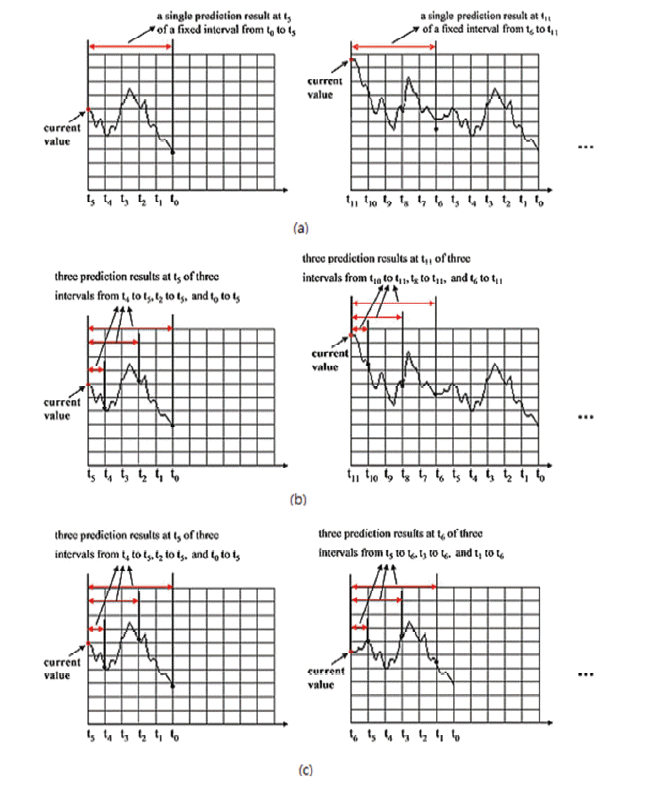##### Fig. 2
The continuous integrated hierarchical temporal memory (CIHTM) network for continuous multi-interval prediction. (a) Structure of CIHTM network for training, (b) structure of CIHTM network for prediction. ZT=Zeta1TopNode, ZF=Zeta1FirstNode, Z1=Zeta1Node.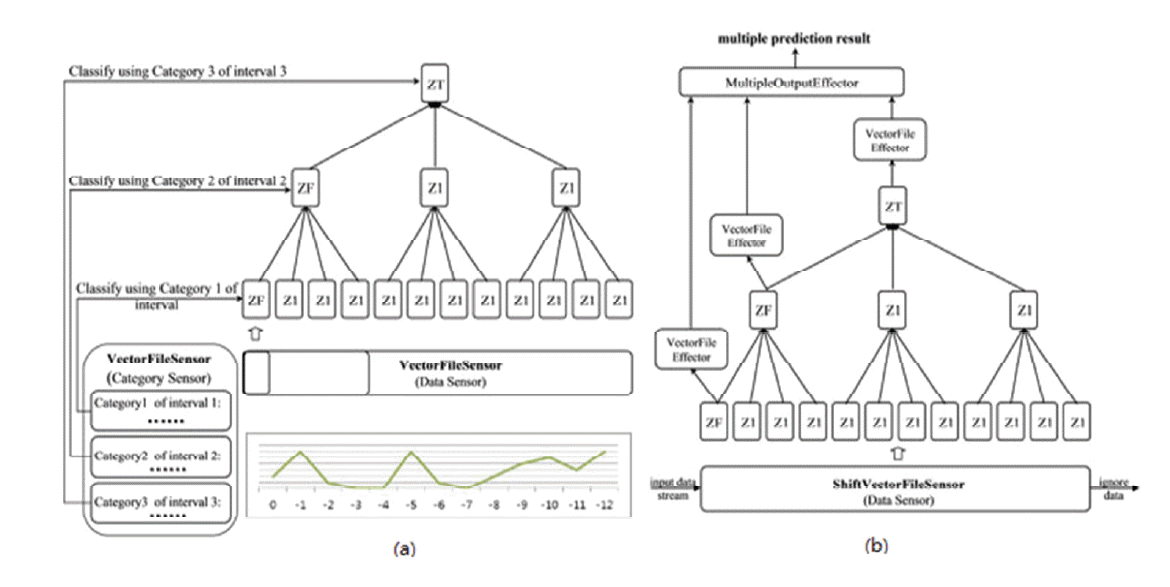##### Fig. 3
Structure of the ZFSQNode.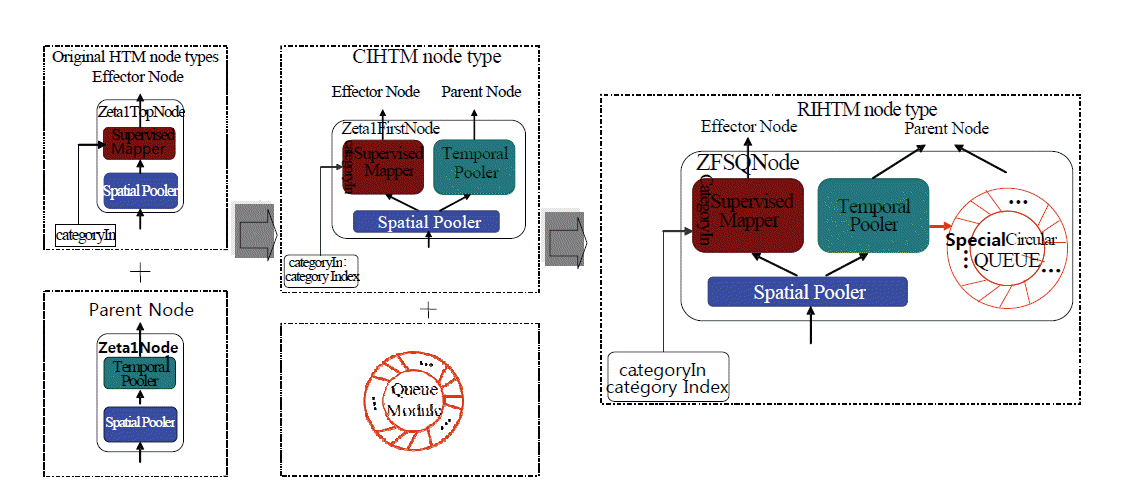##### Fig. 4
An initial specialized circular queue of the ZFSQNode.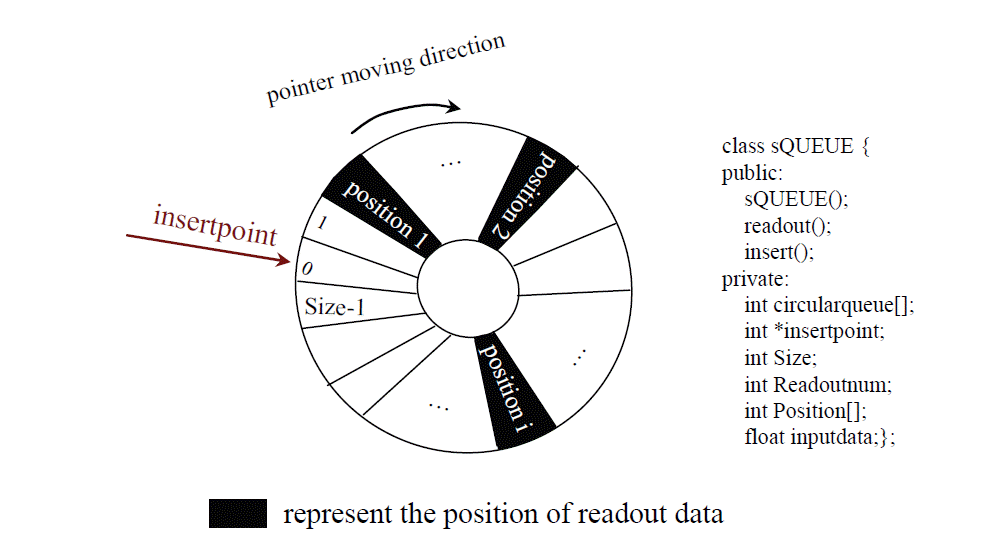##### Fig. 5
Real-time integrated hierarchical temporal memory (RIHTM) network for real-time continuous multi-interval prediction (RCMIP).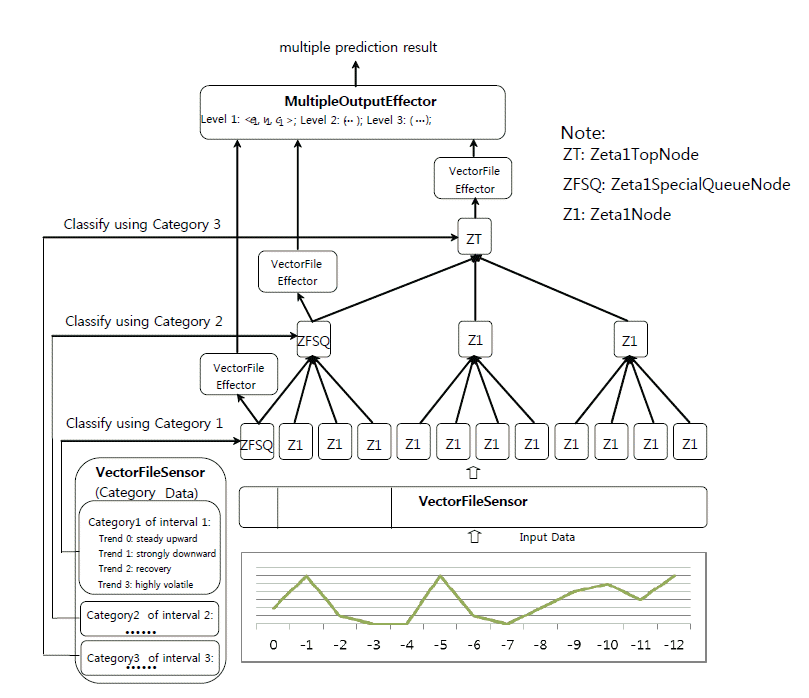##### Fig. 6
Real-time integrated hierarchical temporal memory (RIHTM) network for the training stage. Z1=Zeta1Node, ZT=Zeta1TopNode, ZFSQ=Zeta1FirstSpecializedQueueNode.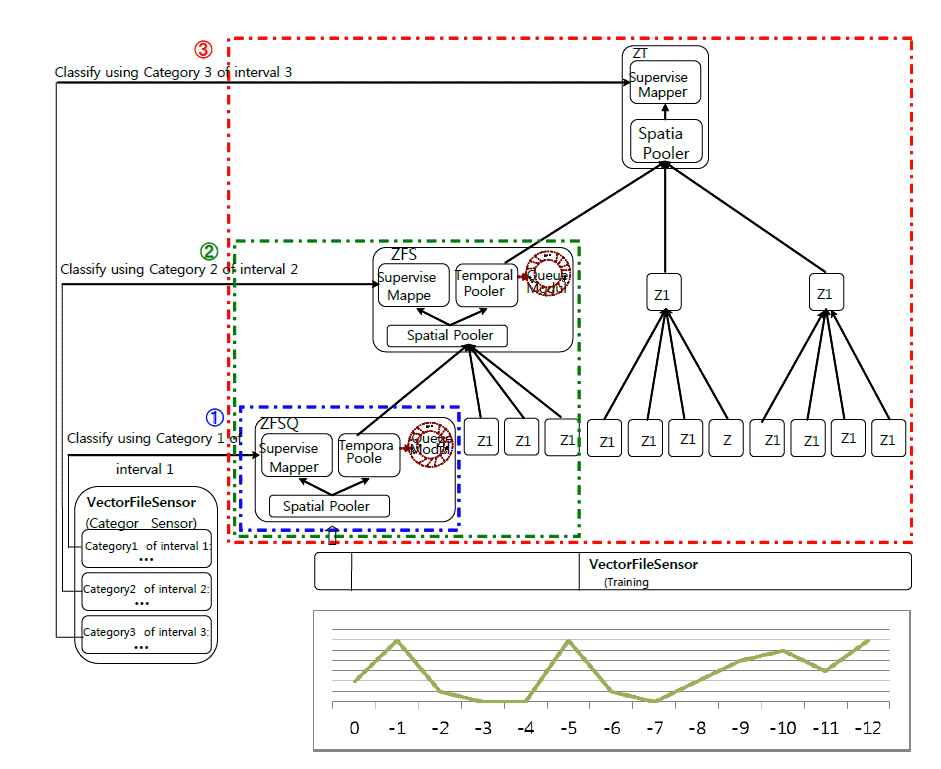##### Fig. 7
The real-time integrated hierarchical temporal memory (RIHTM) network for the prediction stage. ZT=Zeta1TopNode, ZFSQ=Zeta1FirstSpecializedQueueNode.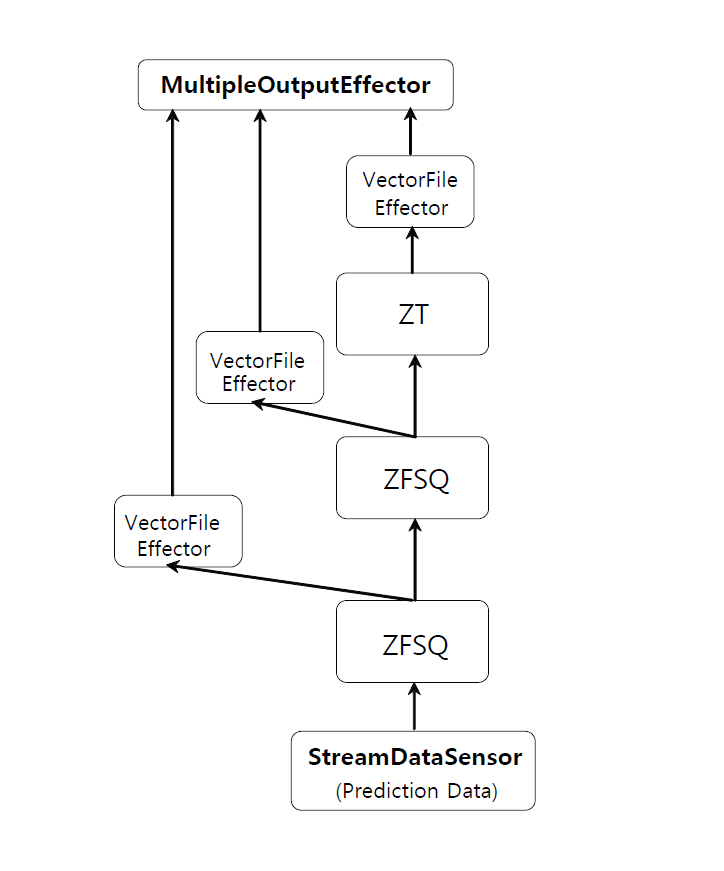##### Fig. 8
Predictions by the real-time integrated hierarchical temporal memory (RIHTM) network in Step 1. ZT=Zeta1TopNode, ZFSQ=Zeta1FirstSpecializedQueueNode, sQUEUE=specialized circular queue.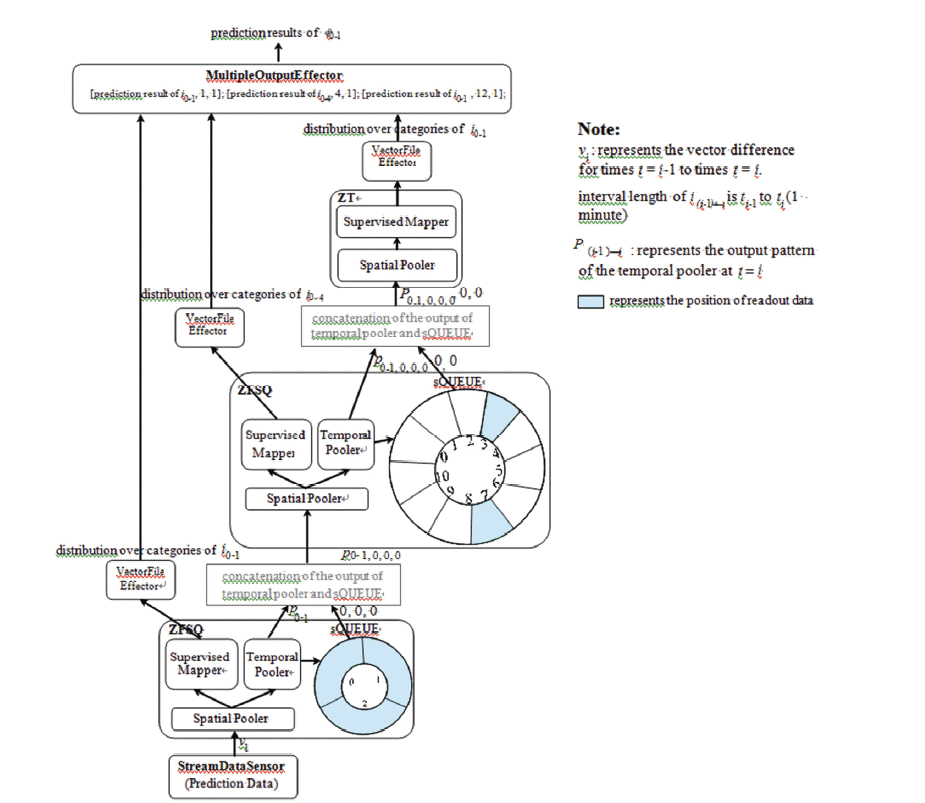##### Fig. 9
Two kinds of networks for data streams. (a) The continuous integrated hierarchical temporal memory (CIMTM) network, (b) the real-time integrated hierarchical temporal memory (RIHTM) network. Z1=Zeta1Node, ZF=Zeta1FirstNode, ZT=Zeta1TopNode, ZFSQ=Zeta1FirstSpecializedQueueNode.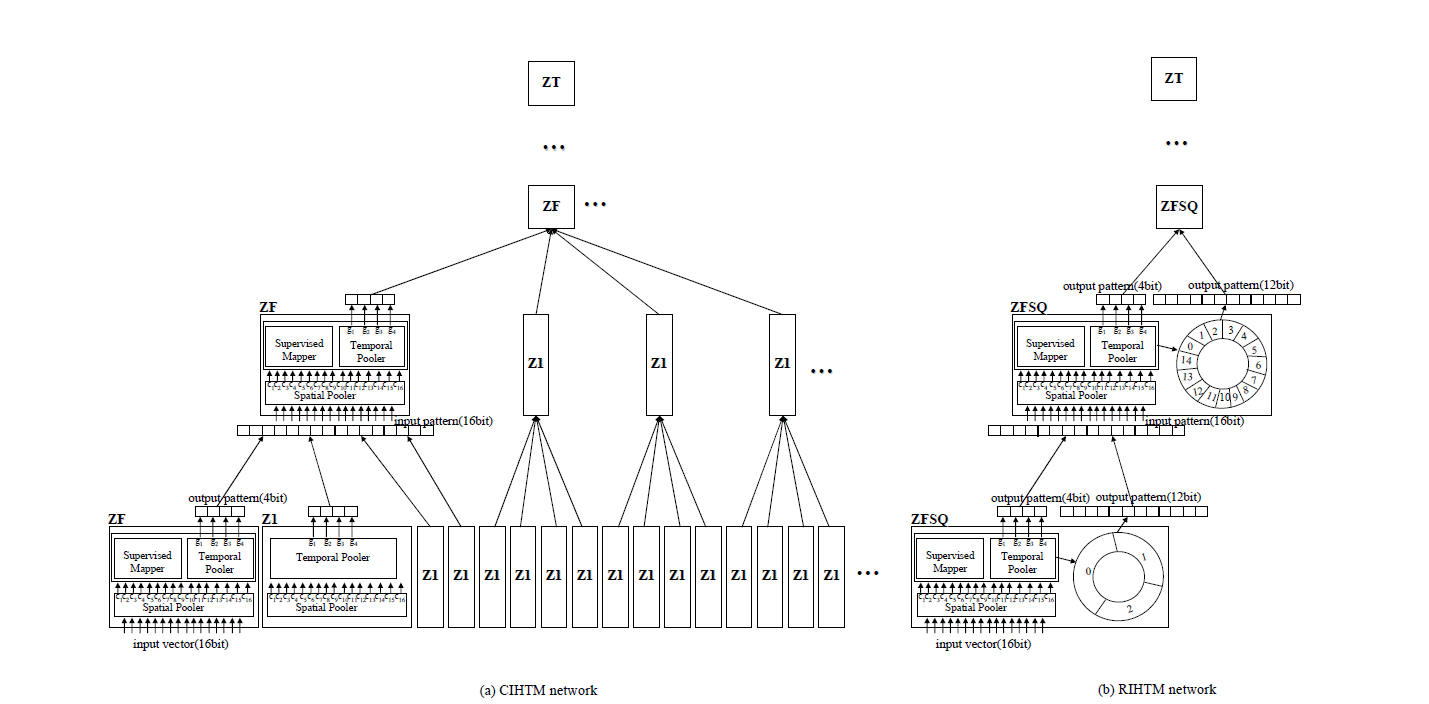##### Fig. 10
Memory consumptions of networks. CIHTM=continuous integrated hierarchical temporal memory, RIHTM=real-time integrated hierarchical temporal memory.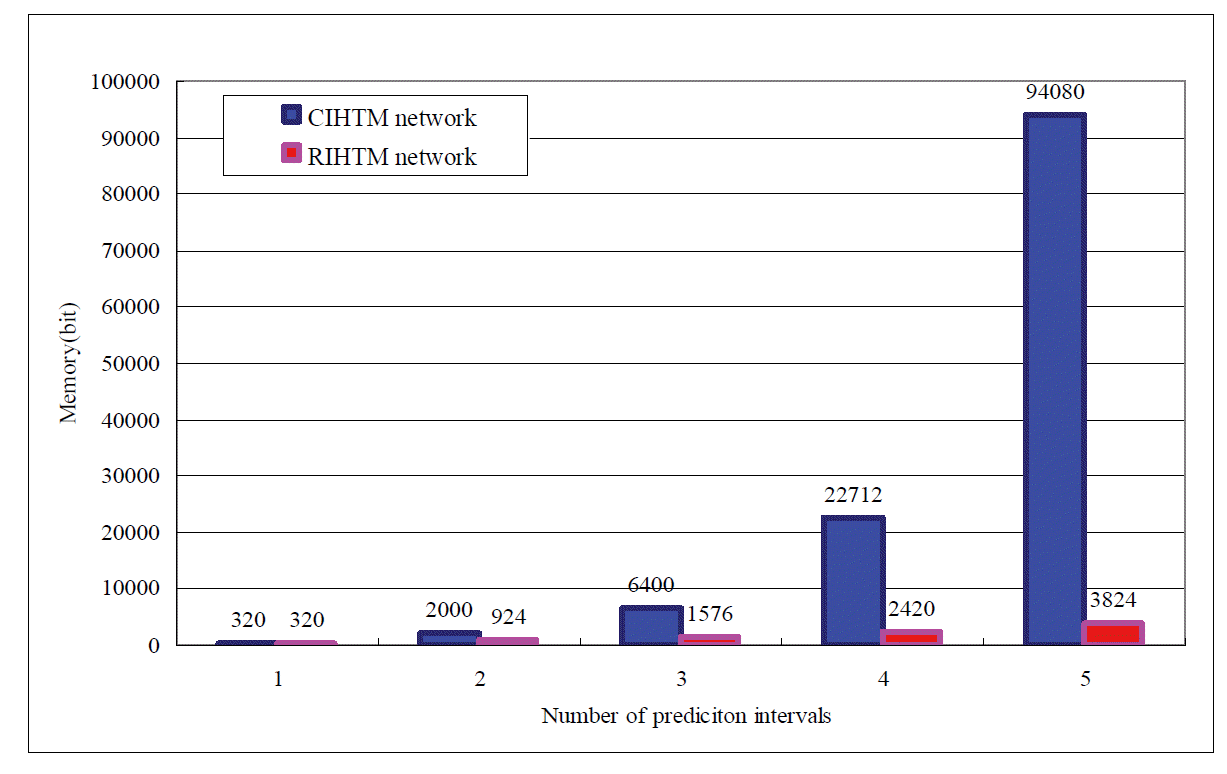##### Fig. 11
Time consumptions of networks. CIHTM=continuous integrated hierarchical temporal memory, RIHTM=real-time integrated hierarchical temporal memory.